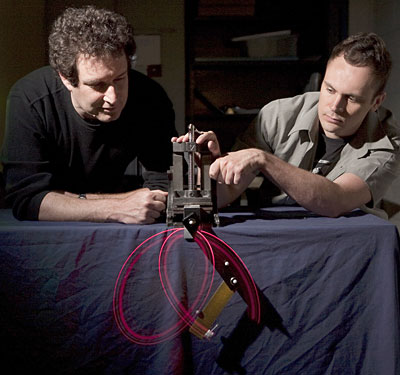# Move over, Newton: Scientifically ignorant computer derives natural laws from raw dataLindsay France/University Photography
Professor Hod Lipson and graduate student Michael Schmidt adjust a double pendulum. Refectors on the pendulum enable motion-tracking software to record position and velocity as the pendulum swings. From this a new computer algorithm can derive equations of motion.

If Isaac Newton had had access to a supercomputer, he'd have had it watch apples fall and let it figure out what that meant. But the computer would have needed to run an algorithm developed by Cornell researchers that can derive natural laws from observed data.

The researchers have taught a computer to find regularities in the natural world that represent natural laws -- without any prior scientific knowledge on the part of the computer. They have tested their method, or algorithm, on simple mechanical systems and believe it could be applied to more complex systems ranging from biology to cosmology and be useful in analyzing the mountains of data generated by modern experiments that use electronic data collection.

The research is described in the April 3 issue of the journal Science (Vol. 323, No. 5924) by Hod Lipson, associate professor of mechanical and aerospace engineering, and graduate student Michael Schmidt, a specialist in computational biology.

Their process begins by taking the derivatives of every variable observed with respect to every other -- a mathematical way of measuring how one quantity changes as another changes. Then the computer creates equations at random using various constants and variables from the data. It tests these against the known derivatives, keeps the equations that come closest to predicting correctly, modifies them at random and tests again, repeating until it literally evolves a set of equations that accurately describe the behavior of the real system.

Technically, the computer does not output equations, but finds "invariants" -- mathematical expressions that remain true all the time, from which human insights can derive equations.

"Even though it looks like it's changing erratically, there is always something deeper there that is always constant," Lipson explained. "That's the hint to the underlying physics. You want something that doesn't change, but the relationship between the variables in it changes in a way that's similar to [what we see in] the real system."

Once the invariants are found, potentially all equations describing the system are available: "All equations regarding a system must fit into and satisfy the invariants," Schmidt said. "But of course we still need a human interpreter to take this step."

The researchers tested the method with apparatus used in freshman physics courses: a spring-loaded linear oscillator, a single pendulum and a double pendulum. Given data on position and velocity over time, the computer found energy laws, and for the pendulum, the law of conservation of momentum. Given acceleration, it produced Newton's second law of motion.

The researchers point out that the computer evolves these laws without any prior knowledge of physics, kinematics or geometry. But evolution takes time. On a parallel computer with 32 processors, simple linear motion could be analyzed in a few minutes, but the complex double pendulum required 30 to 40 hours of computation. The researchers found that seeding the complex pendulum problem with terms from equations for the simple pendulum cut processing time to seven or eight hours. This "bootstrapping," they said, is similar to the way human scientists build on previous work.

Computers will not make scientists obsolete, the researchers conclude. Rather, they said, the computer can take over the grunt work, helping scientists focus quickly on the interesting phenomena and interpret their meaning.

The research was supported by the National Science Foundation.